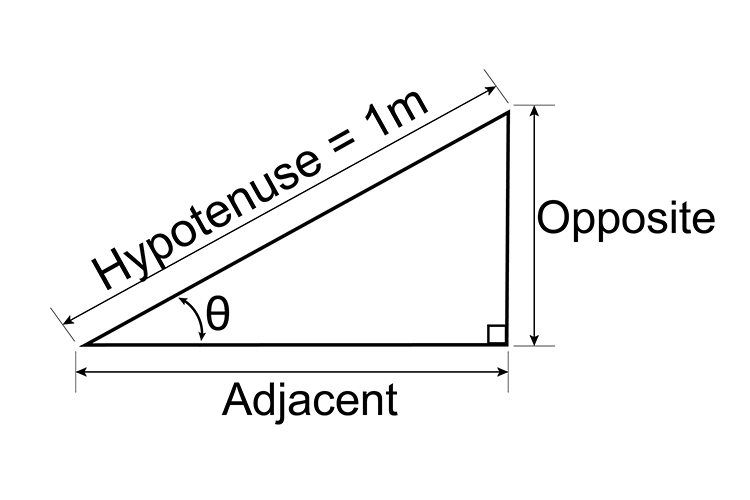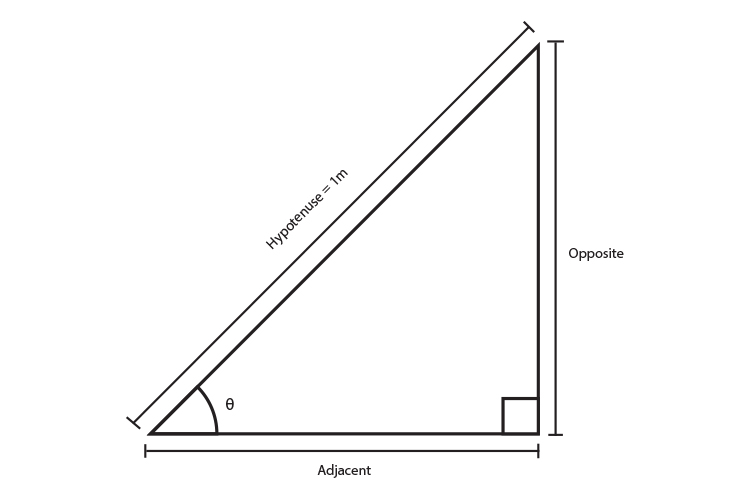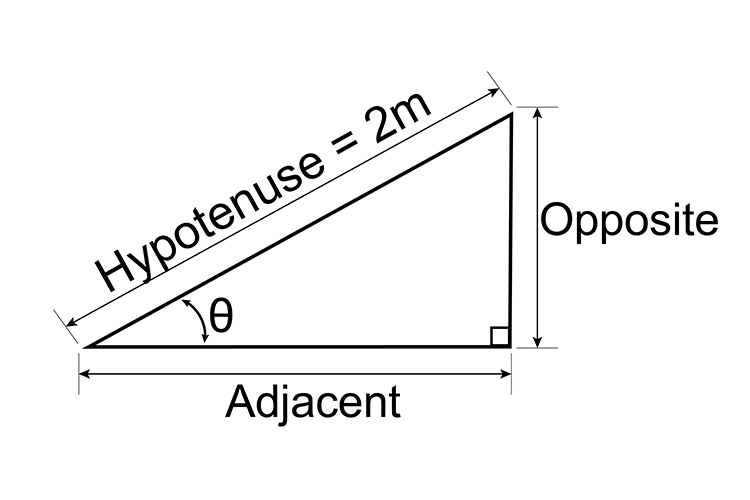# Cosine examples

Example 1

If the hypotenuse is 1 metre long and theta=35^@, how long is the adjacent side?Look up on the table/chart of cosine (angle) for 35^@ or look up cosine 35^@ on a calculator.

 Angle Adjacent distance (or cosine) 35^@ 0.8192 metres

Hypotenuse = 1 metre,

theta=35^@,

Example 2

If the hypotenuse is 1 metre long and theta=45^@, how long is the adjacent side?Look up on the table/chart of cosine (angle) for 45^@ or look up cosine 45^@ on a calculator.

 Angle Adjacent distance (or cosine) 45^@ 0.7071 metres

Hypotenuse = 1 metre,

theta=45^@,

Example 3

If the hypotenuse is 1 metre long, and the adjacent side is 0.8 metres long, what is the angle theta  ?Look up on the table/chart of cosine (angle) for 0.8 metres and find the angle.

 Angle Adjacent distance (or cosine) ? 0.8 metres

The nearest = 36° = 0.809 metres

But on a calculator if you put in 0.8 and press inverse cosin (cos-1), this gives you 36.87° (which is more accurate).

Example 4

If the hypotenuse is 2 metres long and theta=45^@, how long is the adjacent side?Look up on the table/chart of cosine (angle) for 45^@ or look up cosine 45^@ on a calculator.

 Angle Adjacent distance (or cosine) 45^@ 0.7071 metres

But 0.7071 is for a 1m hypotenuse.

For a hypotenuse of 2m the adjacent would be twice as long.

Therefore 0.7071times2 = 1.4142

theta=45^@,